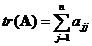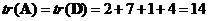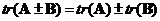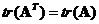## Matrix Trace

Trace of a square matrix is the summation of matrix diagonal entries. Trace of a matrix is also called spur of a square matrix.

Example : Given matrixThe diagonal matrix isThus, the trace isThe interactive program below produces trace of your matrix input. The input matrix is shown back for your feedback. Random Example button will generate random square matrix at random order.

## Properties

Some important properties of the trace of a matrix are

• Trace of a matrix is a linear operation. Trace of a summation or subtraction of two matrices is equal to the summation or subtraction of trace of the matrices, that is• Trace of a matrix transpose is equal to the trace of the original matrix